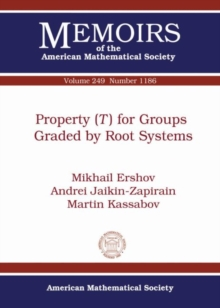Supporting your high street Find out how »
• My Account# Property ($T$) for Groups Graded by Root Systems Paperback / softback

## Part of the Memoirs of the American Mathematical Society series

#### Description

The authors introduce and study the class of groups graded by root systems.

They prove that if $\Phi$ is an irreducible classical root system of rank $\geq 2$ and $G$ is a group graded by $\Phi$, then under certain natural conditions on the grading, the union of the root subgroups is a Kazhdan subset of $G$.

As the main application of this theorem the authors prove that for any reduced irreducible classical root system $\Phi$ of rank $\geq 2$ and a finitely generated commutative ring $R$ with $1$, the Steinberg group ${\mathrm St}_{\Phi}(R)$ and the elementary Chevalley group $\mathbb E_{\Phi}(R)$ have property $(T)$.

They also show that there exists a group with property $(T)$ which maps onto all finite simple groups of Lie type and rank $\geq 2$, thereby providing a unified'' proof of expansion in these groups.

#### Information

• Format: Paperback / softback
• Pages: 135 pages
• Publisher: American Mathematical Society
• Publication Date:
• Category: Groups & group theory
• ISBN: 9781470426040

£71.95

£71.55

on all orders

###### Pick up orders

from local bookshops

£77.50

£76.95

£77.50

£76.95

£77.50

£76.95

£77.50

£76.95